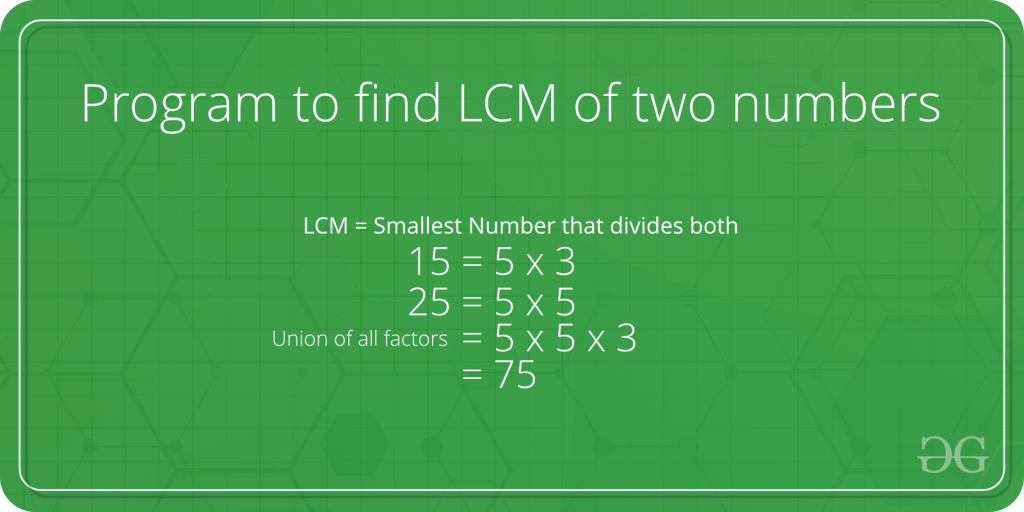# Java Program to Find LCM of Two Numbers

LCM (i.e. Least Common Multiple) is the largest of the two stated numbers that can be divided by both the given numbers.### Example for LCM of Two Numbers

Input: LCM( 15 and 25)
Output: 75

Input: LCM( 3 and 7 )
Output: 21

## Methods to Find LCM

There are certain methods to Find the LCM of two numbers as mentioned below:

• Using if statement
• Using GCD

### 1. Using if statement to Find the LCM of Two Numbers

Using if is a really simple method and also can be said brute force method.

Below is the implementation of the above method:

## Java

 `// Java Program to find ` `// the LCM of two numbers` `import` `java.io.*;`   `// Driver Class` `class` `GFG {` `    ``// main function` `    ``public` `static` `void` `main(String[] args)` `    ``{` `        ``// Numbers` `        ``int` `a = ``15``, b = ``25``;`   `        ``// Checking for the smaller` `        ``// Number between them` `        ``int` `ans = (a > b) ? a : b;`   `        ``// Checking for a smallest number that` `        ``// can de divided by both numbers` `        ``while` `(``true``) {` `            ``if` `(ans % a == ``0` `&& ans % b == ``0``)` `                ``break``;` `            ``ans++;` `        ``}`   `        ``// Printing the Result` `        ``System.out.println(``"LCM of "` `+ a + ``" and "` `+ b` `                           ``+ ``" : "` `+ ans);` `    ``}` `}`

Output

`LCM of 15 and 25 : 75`

### 2. Using Greatest Common Divisor

Below given formula for finding the LCM of two numbers ‘u’ and ‘v’ gives an efficient solution.

```u x v = LCM(u, v) * GCD (u, v)
LCM(u, v) = (u x v) / GCD(u, v)```

Here, GCD is the greatest common divisor.

## Java

 `// Java program to find LCM` `// of two numbers.` `class` `gfg {` `    ``// Gcd of u and v` `    ``// using recursive method` `    ``static` `int` `GCD(``int` `u, ``int` `v)` `    ``{` `        ``if` `(u == ``0``)` `            ``return` `v;` `        ``return` `GCD(v % u, u);` `    ``}`   `    ``// LCM of two numbers` `    ``static` `int` `LCM(``int` `u, ``int` `v)` `    ``{` `        ``return` `(u / GCD(u, v)) * v;` `    ``}`   `    ``// main method` `    ``public` `static` `void` `main(String[] args)` `    ``{` `        ``int` `u = ``25``, v = ``15``;` `        ``System.out.println(``"LCM of "` `+ u + ``" and "` `+ v` `                           ``+ ``" is "` `+ LCM(u, v));` `    ``}` `}`

Output

`LCM of 25 and 15 is 75`

#### Complexity of the above method:

Time Complexity: O(log(min(a,b))
Auxiliary Space: O(log(min(a,b))

Whether you're preparing for your first job interview or aiming to upskill in this ever-evolving tech landscape, GeeksforGeeks Courses are your key to success. We provide top-quality content at affordable prices, all geared towards accelerating your growth in a time-bound manner. Join the millions we've already empowered, and we're here to do the same for you. Don't miss out - check it out now!

Previous
Next